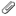#### General Information#

Language: ACTION!
Compiler/Interpreter: ACTION!
Published: ANTIC Power Computing, September 1985

## 8 QUEENS ACTION!#

### 92 chess solutions in 40 seconds#

Lightning fast ACTION! solution to "The Eight Queens Problem" from the April, 1985 Antic. Requires the ACTION! programming language cassette from Optimized Systems Software. Works on all 8-bit Atari computers of any memory size, with disk or cassette. Disk subscribers: You can use this program without ACTION! Select the "L" option from DOS 2 for the file, QUEEN EXE.

In line with Antic's long-held belief that our published programs are part of a two-way communications process with readers of the magazine, many Antic programming take-aparts conclude with suggestions for possible enhancements that an ambitious programmer might make in the listings.

But Dave Oblad took it as a personal challenge when he saw Angelo Giambra's "The Eight Queens Problem" in the April, 1985 Antic and read our final comment: "For a real challenge, you might want to try modifying the program so that only the 12 unique solutions are found."

(The original April article showed that there are 92 possible ways to arrange eight queens on a chessboard so that none of them threatens another. As the most powerful chess piece, a queen can attack for any distance along any straight line.)

In Dave's letter to Antic, he wrote, "I spent the next two days cranking away at my Atari in the ACTION! language, which is much faster than BASIC. My algorithm solves and displays all 92 general solutions in approximately 40 seconds-and finds the 12 unique solutions in 30 seconds."

```; 8-QUEENS SOLUTION
; (c) 1985, ANTIC PUBLISHING

BYTE ARRAY T(96),P(8),I(8),O(8),M(8)
BYTE A,B,C,D,X,Y,L1,L2,L3,OPT=53279

PROC SEARCH()
FOR X=0 TO D
DO
Y=X*8 B=1
FOR A=0 TO 7
DO
IF T(Y+A)#O(A) THEN B=0 FI
OD
IF B=1 THEN RETURN FI
OD
RETURN

PROC ROTATE()
FOR A=0 TO 7
DO
B=7-O(A) M(B)=A
OD
FOR A=0 TO 7
DO
O(A)=M(A)
OD
RETURN

PROC TEST()
FOR A=0 TO 7
DO O(A)=P(A) OD
FOR L1=0 TO 1
DO
FOR L2=0 TO 1
DO
FOR L3=0 TO 3
DO
SEARCH()
IF B=1 THEN RETURN FI
ROTATE()
OD
FOR A=0 TO 7
DO M(A)=O(A) OD
FOR A=0 TO 7
DO O(7-A)=M(A) OD
OD
FOR A=0 TO 7
DO O(A)=7-O(A) OD
OD
B=0
RETURN

PROC KEEP()
X=D*8
FOR A=0 TO 7
DO T(X+A)=P(A) OD
RETURN

PROC DISPLAY()
;REMOVE 5 SEMI-COLONS BELOW
;FOR UNIQUE SOLUTIONS ONLY!

;IF D#0 THEN TEST()
; IF B=1 THEN RETURN
;  ELSE KEEP()
; FI
;FI

FOR Y=0 TO 7
DO
FOR X=0 TO 7
DO
POSITION(X+15,Y+8)
IF P(Y)=X THEN PRINT("Q")
ELSE PRINT("+") FI
OD
OD
POSITION(18,18)
D==+1 PRINTB(D)
RETURN

PROC TRY()
FOR Y=0 TO 6
DO
FOR X=Y+1 TO 7
DO
A=P(X)-P(Y) B=X-Y
IF A>7 THEN A=255-A+1 FI
IF A=B THEN RETURN FI
OD
OD
DISPLAY()
RETURN

PROC SWAP()
C=0 I(C)==+1
WHILE I(C)=C+2
DO›   I(C)=0 C==+1 I(C)==+1
IF C<7 THEN
FOR B=0 TO C
DO
A=P(B) P(B)=P(B+1) P(B+1)=A
OD
FI
OD
A=P(0) P(0)=P(1) P(1)=A
RETURN

PROC MAIN()
BYTE CONSOLE=53279

DO
GRAPHICS(0) POKE(752,1)
POSITION(8,0)
PRINTE("  8-QUEENS SOLUTIONS")
FOR A=0 TO 7 DO P(A)=A I(A)=0 OD
FOR A=0 TO 96 DO T(A)=0 OD
D=0
DO
TRY() SWAP()
FOR A=0 TO 7
DO
IF A#P(A) THEN EXIT FI
OD
IF A=8 OR OPT#7 THEN EXIT FI
OD
IF A=8 THEN POSITION(15,20)
PRINTE("COMPLETE")
PUTE()
PRINTE("PRESS  START  TO RE-RUN")
FI
DO
UNTIL CONSOLE < 7
OD
OD
RETURN
```

PDF: Eight Queens/8queensaction.PDFDJVU: Eight Queens/8queensaction.djvuOnly authorized users are allowed to upload new attachments.

### List of attachments

Kind Attachment Name Size Version Date Modified Author Change note
pdf
8queensaction.PDF 372.8 kB 1 13-Mar-2010 15:34 Carsten Strotmann
djvu
8queensaction.djvu 223.5 kB 1 13-Mar-2010 15:34 Carsten Strotmann
gif
8queensaction.gif 18.1 kB 1 13-Mar-2010 15:34 Carsten Strotmann
« This page (revision-9) was last changed on 03-Feb-2023 16:21 by Gromit
G’day (anonymous guest) My Prefs
Articles
Hardware
Software
Applications
Memory Map Topic list
MicroUSB
AllNews
FAQ
Index
Languages
Code

What's New?

Voice and Data Cassettes
Atari Program Exchange (APX)
Atari Learning System Software
PLATO (Programmed Logic for Automated Teaching Operation)
Podcasts
Official country specific Atari software

Thanks
Rarity 10
Statistics
All content in the Wiki is licensed under Creative Commons Share Alike License, unless otherwise noted.